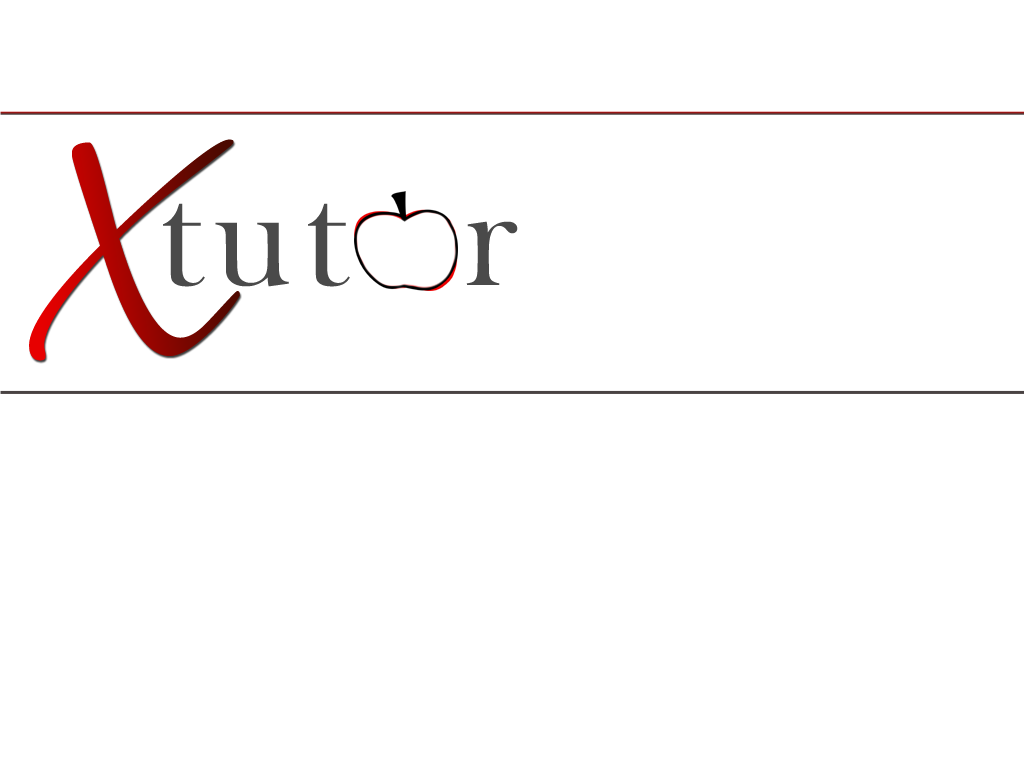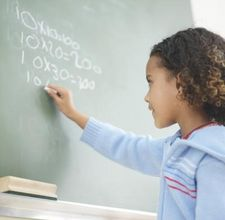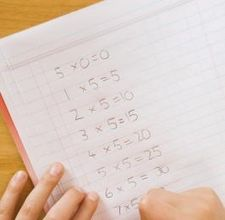Elementary Math Numbers and operations: Introducing and counting numbers one through ten and then using these numbers to add, subtract, multiply and divide. Once a solid foundation is built on the basics then decimals and fractions are used to demonstrate parts of unit wholes, as parts of a collection, and as locations on number line.Measurements: This concept is first introduced to students through money and time. Once they have mastered these concepts, teachers introduce capacity, length, and weight measurements. By this time students should be able to do basic converting and comparing of US and metric units. Geometry: Identifying and describing of two and three dimensional shapes. Once taught to characterize and analyze by said properties. Algebra: Elementary students are able to sort and classify objects by size, number, etc. while representing and analyzing patterns and functions, using words, tables, and graphs.Data Analysis and Probability: Students are first introduced to data analysis and probability by exploring their personal surroundings and then asking questions about those surroundings. Their gathering skills are then enhanced through various data collection methods which in turn is used to sort through the differences in categorical and numerical data.